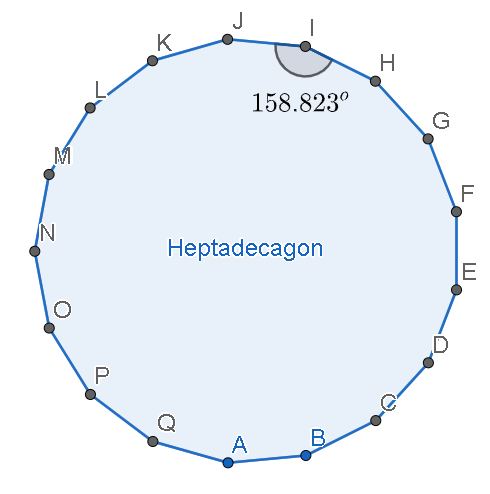Courses
Courses for Kids
Free study material
Offline Centres
MoreLast updated date: 02nd Dec 2023
Total views: 280.8k
Views today: 4.80k

# What is the internal angle of a regular 17-sided polygon?Verified
280.8k+ views
Hint: To find the internal angle of a regular 17-sided polygon, we will use the formula for the sum of interior angles of a polygon which is given by $S=180\left( n-2 \right)\text{ degrees}$ . We will substitute $n=17$ in this formula and find the sum. The measure of one interior angle can be obtained by dividing this sum of the interior angles by the number of sides of the polygon.

Complete step by step solution:
We have to find the internal angle of a regular 17-sided polygon. We know that an interior angle of a polygon is an angle formed inside the two adjacent sides of a polygon. Or we can say that they are the angle measures at the interior part of a polygon.
We know that the sum of interior angles of a polygon is given by the formula
$S=180\left( n-2 \right)\text{ degrees}$
where, n is the number of sides of the polygon.
We are given a 17 sided polygon. Therefore, $n=17$ . Let us substitute this value in the formula for the sum of the interior angles.
$\Rightarrow S=180\left( 17-2 \right)$
We have subtract 2 from 17.
$\Rightarrow S=180\times 15$
We have to multiply 180 and 15.
$\Rightarrow S=2700{}^\circ$
Therefore, the sum of all the angles in a 17 sided polygon is $2700{}^\circ$ .
Therefore, the measure of 1 interior angle can be obtained by dividing the sum of all the interior angles by the number of sides.
$\Rightarrow$ Measure of one interior angle $=\dfrac{S}{n}$
Let us substitute the values in the above formula.
$\Rightarrow$ Measure of one interior angle $=\dfrac{2700{}^\circ }{17}$
$\Rightarrow$ Measure of one interior angle $=158.823{}^\circ$
The figure for a regular 17 sided-polygon called a heptadecagon is shown below.Therefore, the internal angle of a regular 17-sided polygon is $158.823{}^\circ$ .

Note: Students must note the formula for the sum of the interior angles of a polygon remains the same for a regular and an irregular polygon but the individual angles vary in the latter. The sum of interior angles of a polygon is always a constant value. A polygon that does not have all sides equal and all angles equal. Is called irregular polygon in which a regular polygon will have all equal sides and equal angles. Students must never forget to put the symbol of degree after finding the measure of the angles.